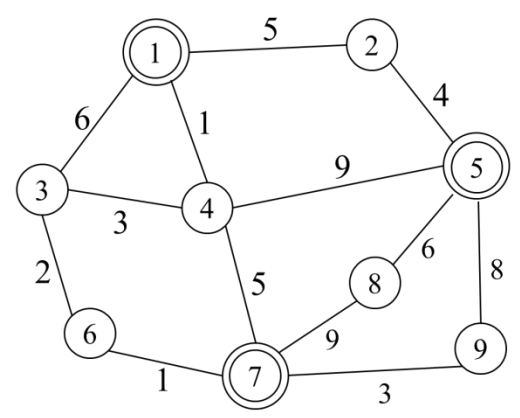시간 제한메모리 제한제출정답맞힌 사람정답 비율
2 초 512 MB0000.000%

## 문제

An undirected weighted graph G is given and G is connected, that is, arbitrary two vertices in G must be connected by a path. Three robots are exploring G through edges. Here the weight of each edge means the time to be spent when a robot passes through it. The speeds of all the robots are identical and at least two robots may be passed through a same edge at a time. At some instant of the explorations of the robots, all of them should get together at a vertex to share their information. It is called a rendezvous.

Initially, three robots lie on specified vertices. Of course, at least two robots may be located on an identical vertex. Also all the three robots start to move simultaneously. We wish to know the minimum time needed to fulfill the first rendezvous.Figure L.1

For example, initially, three robots are located on the vertices 1, 5, and 7 in Figure L.1. A movement of the robot on the vertex 1 into the vertex 9 requires at least 9 time units. Also it takes at least 8 and 3 time units, respectively that the robots on the vertices 5 and 7 travel into the vertex 9. So the rendezvous at the vertex 9 requires at least 9 time units and it is the minimum time needed for the first rendezvous. Of course, the first rendezvous happens either at the vertex 1 or 4 and it also requires the minimum time of 9.

Given a weighted and connected graph G and the initial locations of three robots, write a program to find the minimum time needed to fulfill the first rendezvous.

## 입력

Your program is to read from standard input. The input starts with a line containing two integers, N and M (1 ≤ N ≤ 20,000, N − 1 ≤ M ≤ 100,000), where N and M are the numbers of vertices and edges of G, respectively. The vertices of G are represented by 1, 2, … , N. In each of the following M lines, three integers a, b, and t (1 ≤ abN, 1 ≤ t ≤ 10,000) are given, where an edge connects the two vertices a and b, and its weight is t. The last (M + 2)-th line contains three integers u, v, and w, which are the initial locations of three robots (1 ≤ u, v, wN). Of course, it is possible that at least two of the three robots are initially located on an identical vertex.

## 출력

Your program is to write to standard output. Print exactly one line which contains the minimum time needed to fulfill the first rendezvous.

## 예제 입력 1

4 6
1 2 8
3 2 6
3 1 1
1 4 10
4 2 2
3 4 3
1 1 2


## 예제 출력 1

4


## 예제 입력 2

9 13
1 2 5
3 1 6
1 4 1
2 5 4
3 4 3
5 4 9
6 3 2
4 7 5
8 5 6
7 8 9
5 9 8
7 6 1
7 9 3
1 5 7


## 예제 출력 2

9